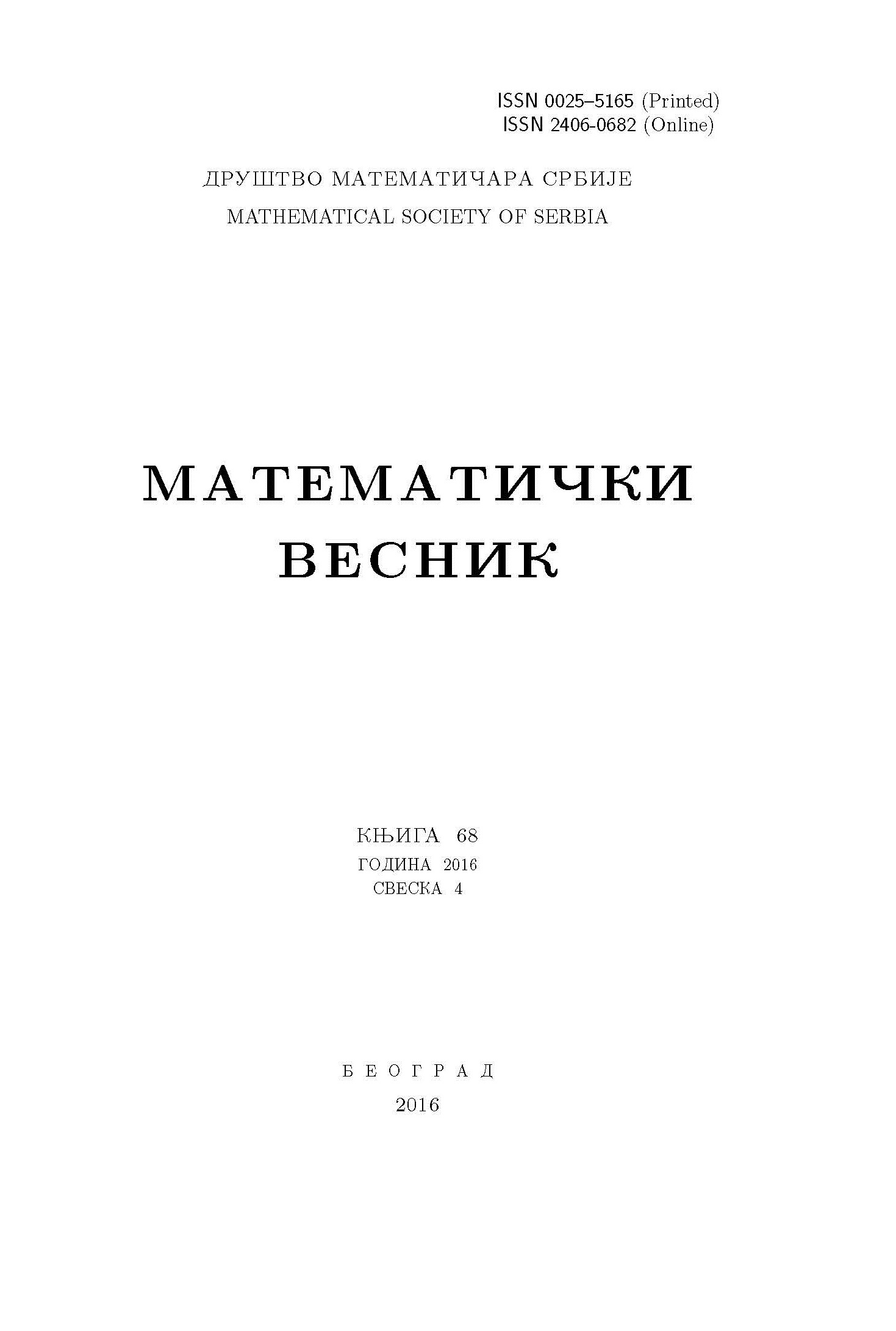﻿ Matematički Vesnik ﻿
 MATEMATIČKI VESNIK МАТЕМАТИЧКИ ВЕСНИКON THE SUBTRACTIVE SUBSEMIMODULE-BASED GRAPH OF SEMIMODULES F. Esmaeili Khalil Saraei, S. Raminfar AbstractLet $M$ be a semimodule over a commutative semiring $R$ and $K$ be a subtractive subsemimodule of $M$ with $K^{*}=K\setminus \{0\}$. The subtractive subsemimodule-based graph of $M$ is defined as the simple undirected graph $\Omega=\Gamma_{K^{*}}(M)$ with vertex set $V(\Omega)=\{v\in M\setminus K : v+v'\in K^{*} \textrm{ for some }v\neq v'\in M\setminus K\}$, and two distinct vertices $m$ and $n$ are adjacent if and only if $m+n\in K^{*}$. In this paper, we study the interplay between semimodule properties and the properties of the graph. Among other results, we compute the diameter and the girth of $\Gamma_{K^{*}}(M)$.Keywords: Semiring; subtractive subsemimodule; partitioning subsemimodule. MSC: 16Y60, 05C753 Pages:  1$-$10

﻿